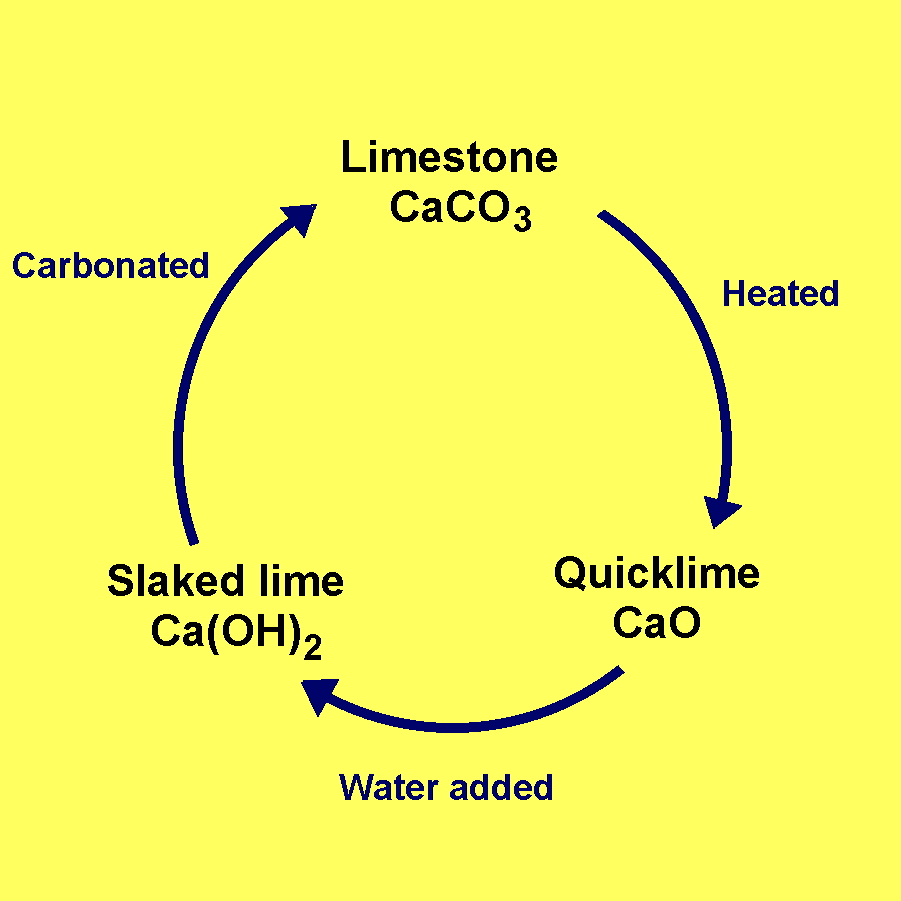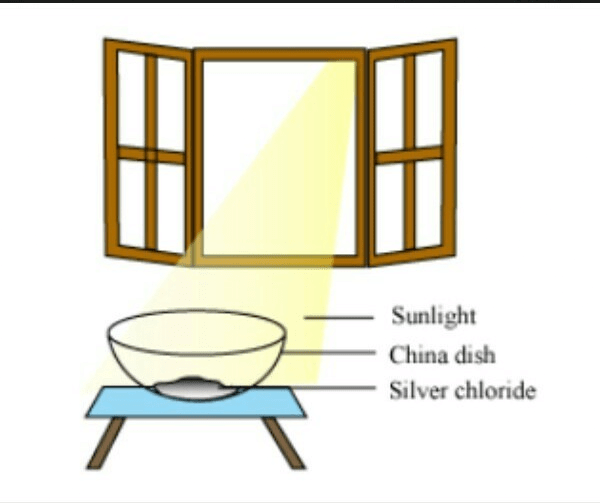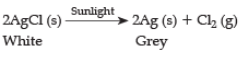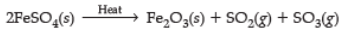Worksheet: Chemical Reactions & Equations - 1

# Worksheet: Chemical Reactions & Equations - 1 Notes | Study Science Class 10 - Class 10

## Document Description: Worksheet: Chemical Reactions & Equations - 1 for Class 10 2022 is part of Science Class 10 preparation. The notes and questions for Worksheet: Chemical Reactions & Equations - 1 have been prepared according to the Class 10 exam syllabus. Information about Worksheet: Chemical Reactions & Equations - 1 covers topics like and Worksheet: Chemical Reactions & Equations - 1 Example, for Class 10 2022 Exam. Find important definitions, questions, notes, meanings, examples, exercises and tests below for Worksheet: Chemical Reactions & Equations - 1.

Introduction of Worksheet: Chemical Reactions & Equations - 1 in English is available as part of our Science Class 10 for Class 10 & Worksheet: Chemical Reactions & Equations - 1 in Hindi for Science Class 10 course. Download more important topics related with notes, lectures and mock test series for Class 10 Exam by signing up for free. Class 10: Worksheet: Chemical Reactions & Equations - 1 Notes | Study Science Class 10 - Class 10
 1 Crore+ students have signed up on EduRev. Have you?

Q1. Which of the following is not a physical change? (1 Mark)
(A) Boiling of water to give water vapour
(B) Melting of ice to give water
(C) Dissolution of salt in water
(D) Combustion of liquefied petroleum gas (LPG)
Ans: Option D
Explanation: During the combustion of liquefied petroleum gas (LPG), it forms CO2 and H2O (chemical change), while in other cases, there's just a change of state (or phycial change).

Q2. Read the given passage and answer the following questions.
In the following chemical reaction, ‘‘zinc oxide reacts with carbon to produce zinc
metal and carbon monoxide.’’
ZnO + C → Zn + CO

(a) The substance getting oxidized is ________ and the one getting reduced is ________.  (1 Marks)
(b) State the reason for choosing the substances (a). (1 Marks)
(c) Name the type of reaction.  (1 Marks)
(d) Give another example of a similar type of reaction. (1 Marks)
Ans. (a) C is getting oxidized to CO, ZnO is getting reduced to Zn.
(b) As carbon is gaining oxygen and ZnO is losing oxygen.
(c) It is a redox reaction or oxidation and reduction reaction.
(d) CuO + H2 → Cu + H2O

Q3. Which one is a chemical change–rusting of iron or melting of iron?  (1 Marks)
Ans. Rusting of iron

Q4. What happens when quick lime is added to water?  (1 Marks)
Ans. Quick lime reacts with water vigorously to produce slaked lime and a large amount of heat i.e. CaO(s) (Quick lime)+ H2O (l) → Ca(OH)2 (Slaked lime) + HeatQ5. Identify the type of reactions taking place in each of the following cases and write the balanced chemical equation for the reactions.
(a) Zinc reacts with silver nitrate to produce zinc nitrate and silver.
(b) Potassium iodide reacts with lead nitrate to produce potassium nitrate and lead iodide.  (3 Marks)(CBSE Marking Scheme, 2019)
Ans. (a) Displacement reaction i.e. Zn + 2AgNO3 → Zn (NO3)2 + 2Ag
(b) Double displacement reaction  i.e. 2KI + Pb(NO3)2 → PbI2 + 2KNO3

Q6. (i) Define corrosion. What name is given to the corrosion of iron?
(ii) Name the color of the coating formed on silver and copper articles when exposed to air.
(iii) List two damages caused by corrosion and suggest how corrosion can be prevented.  (5 Marks)
Ans. (i) Corrosion is a process in which metals are deteriorated by air, moisture, chemicals, etc. Corrosion of iron is Rusting.
(ii) Silver - black, copper - green.
(iii) (a) Car bodies, bridges, railing, etc. (Any two)
(b) Painting, alloying, greasing, etc.  (Any two)

Q7. Identify the type of chemical reaction in the following statements and define each of them :
(i) Digestion of food in our body
(ii) Rusting of iron
(iii) Heating of manganese dioxide with aluminum powder.
(iv) Blue color of copper sulphate solution disappears when iron filings are added to it.
(v) Dilute hydrochloric acid is added to sodium hydroxide to form sodium chloride and water.  (5 Marks)

Ans. (i) Decomposition Reaction: Carbohydrates are broken down to form glucose.
(ii) Oxidation Reaction: When an iron object is left in moist air for a considerable time, it gets covered with a red-brown flaky substance called rust.
(iii) Displacement reaction: More reactive metal displaces less reactive metal from its salt solution.
(iv) Displacement reaction: More reactive metal displaces less reactive metal from its salt solution.
(v) Double displacement reaction: Reaction in which two compounds react by exchanging ions to form two new compounds.  (CBSE Marking Scheme, 2016)

Q8.  What happens when :
(i) Dilute hydrochloric acid is added to solid sodium carbonate.
(ii) Quicklime is treated with water.
(iii) Sodium chloride solution is added to the lead nitrate solution.
Also, write the chemical equation in each case.   (3 Marks)
Ans.
(i) Na2CO3(s) + 2HCl(aq) → 2NaCl(aq) + H2O(l) + CO2(g)
(ii) CaO(s)  + H2O(l) → Ca(OH)2(aq) + Heat
(iii) Pb(NO3)2(aq) + 2NaCl(aq) → PbCl2(s) + 2NaNO3(aq)

Q9.The following diagram displays a chemical reaction. Observe and answer the following questions:(a) The type of chemical reaction that will take place is
(i) Photochemical decomposition
(ii)  Displacement reaction
(iii) Reduction reaction
(iv) Combination reaction   (1 Marks)
(b) What happens to the silver chloride? (1 Marks)
(c) Write the chemical equation of the reaction involved. (1 Marks)
(d) Mention one commercial use of this salt. (1 Marks)
Ans.
(a) (i) Photochemical decomposition
(b) White silver chloride changes to grey as it decomposes to silver and chlorine in the presence of sunlight.
(c)(d) Black and white photography.

Question for Worksheet: Chemical Reactions & Equations - 1
Try yourself:Q10. A dilute ferrous sulphate solution was gradually added to the beaker containing acidified permanganate solution. The light purple colour of the solution fades and finally disappears. Which of the following is the correct explanation for the observation?

Q11. When a copper wire was left in silver nitrate solution for some time, it was observed that the solution turned bluish-green.
(i) Explain the observation.
(ii) Write the balanced chemical equation to represent the change.   (3 Marks)
Ans.
(i) Copper is more reactive than silver. Hence, when the copper wire is dipped in silver nitrate solution, it displaces silver from AgNO3 solution forming copper nitrate, which is bluish-green in color.

(ii) Cu + 2AgNO3 → Cu(NO3)2 + 2Ag

Q12. (i) Solution of a substance ‘X’ is used for testing carbon dioxide. Write the equation of the reaction of ‘X’ with carbon dioxide.
(ii) How is ‘X’ obtained? Write chemical equations.  (3 Marks)
Ans.
(i) Substance X-Calcium Hydroxide.
Ca(OH)2(aq) + CO2(g) → CaCO3(s) + H2O(l)

CaCO3(s)are white precipitates formed during the reaction.
(ii) Calcium hydroxide is obtained by reaction of calcium oxide and water.
CaO(s) + H2O(l) → Ca(OH)2(aq) + Heat

Q.13. Directions: In the following questions, a statement of assertion (A) is followed by a reason (R).

Assertion (A): Carbon dioxide turns lime water milky.

Reason (R): Carbon dioxide sullies the water.

Mark the correct choice as:

(A) Both assertion (a) and reason (R) are true and reason (R) is the correct explanation of assertion (a).
(B) Both assertion (a) and reason (R) are true but reason (R) is not the correct explanation of assertion (a).
(C) Assertion (a) is true but reason (R) is false.
(D) Assertion (a) is false but reason (R) is true.
Ans: Option C
Explanation: Carbon dioxide reacts with lime water (calcium hydroxide) to form a milky precipitate of calcium carbonate.

Question for Worksheet: Chemical Reactions & Equations - 1
Try yourself:Assertion (A): A chemical reaction becomes faster at higher temperatures.
Reason (R): At higher temperatures, molecular motion becomes more rapid.

Q14. Name and state the law kept in mind while we balance a chemical equation.  (1 Marks)
Ans.
Law of conservation of mass: Mass can neither be created nor destroyed during a chemical reaction.

Q15. 2 g of ferrous sulphate crystals are heated in a dry boiling tube. Answer the following :
(i) List any two observations.
(ii) Name the type of chemical reaction taking place.
(iii) Write the chemical equation of the reaction.  (3 Marks)

Ans. (i) Two observations are :
(a) Change in state and color.
(b) Evolution of gas
(ii) Decomposition reaction
(iii)The document Worksheet: Chemical Reactions & Equations - 1 Notes | Study Science Class 10 - Class 10 is a part of the Class 10 Course Science Class 10.
All you need of Class 10 at this link: Class 10

## Science Class 10

83 videos|341 docs|162 tests
 Use Code STAYHOME200 and get INR 200 additional OFF

## Science Class 10

83 videos|341 docs|162 tests

Track your progress, build streaks, highlight & save important lessons and more!

,

,

,

,

,

,

,

,

,

,

,

,

,

,

,

,

,

,

,

,

,

;